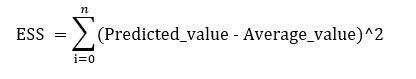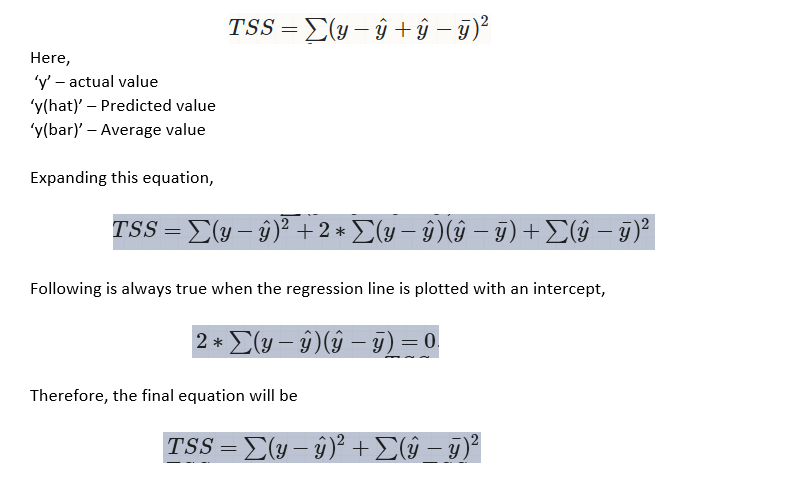# Is R-Square value always between 0 to 1?

R-square value gives the measure of how much variance is explained by model. For a given set of points, the default regression line with minimum sum of square is the horizontal line that passes through the mean. This horizontal regression line denotes that there is no information that can be obtained from the data. If a model can not be designed better, at least it can get into the mean value yielding the minimum square error.

R-Square value can be defined using three other errors terms.

1. Residual Sum of Square (RSS)

It is the summation (for all the data points) of square of difference between the actual and the predicted value.

2. Total Sum of Squares (TSS)

It is the summation (all data points) of square of difference between actual output and average value ‘Y(bar)’Figure 3: Total Sum of Square Formula

3. Explained Sum of Squares (ESS)

It is the summation (for all the data points) of square of difference between the predicted and the average value ‘Y(bar)’.Figure 4: Explained Sum of Square Error Formula

Adding and Subtracting (predicted_value) from TSS, we getFigure 5: Exploring TSS formula in depth

These two terms are ESS and RSS and the equation becomes,

TSS = RSS + ESS

Dividing both the sides by ‘TSS’

TSS/TSS = (RSS/TSS) + (ESS/TSS)

1 — (RSS/TSS) = (ESS/TSS) — — — — — — equation 1

The formula for ‘R-Square’ is,

R2 = 1 — (RSS/TSS) — — — — — — — — — — equation2

On examining the equation 1 and 2, it can be observed that when regression line is plotted with intercept, equation 2 can be replaced by (ESS/TSS). From this equation, it can be inferred that R2 can have maximum value of ‘1’. But minimum value can below 0 and its explanation is given below. Reiterating the points,

• If regression is not done, then horizontal line (average of output) gives the least sum of square errors.
• Regression is done to obtain a line which has error less than the one produced by the horizontal line.
• If the regression is perfect, then regression sum of squares will be zero giving R2 value ‘1’.

But, when we do not specify intercept the below term will not be equal to zero.